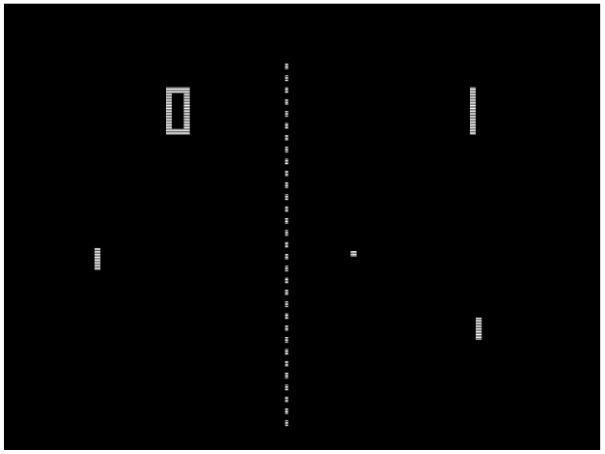# Applications of Calculus: Physics Engines in Video GamesPhysics engines allow video game characters to move in realistic virtual environments, so that if your character throws or jumps or drives a car off a cliff, the result will mimic reality. To perform this task, the physics engine periodically updates the locations of objects, such that the trajectories of objects follow physical laws.

For early games like pong, the physical laws were simple. In pong, the ball travels with a constant velocity $v$ at an angle $\theta,$ and it deflects off paddles such that its incoming angle is equal to its outgoing angle.

The velocity affects the ball’s $x$ and $y$ positions as follows:

\begin{align*} \frac{dx}{dt} &= v \cos (\theta) \\[5pt] \frac{dy}{dt} &= v \sin (\theta) \end{align*}

Using differentials, we write this as

\begin{align*} dx &= v \cos (\theta) dt \\[5pt] dy &= v \sin (\theta) dt \end{align*}

The updates for a ball’s motion across the grid, then, are

\begin{align*} x &\rightarrow x + (v \cos \theta) \Delta t \\[5pt] y &\rightarrow y + (v \sin \theta) \Delta t \end{align*}

where $\Delta t$ is the timestep (the fraction of a second to be simulated) and $\theta$ is updated according to

\begin{align*} \theta \rightarrow 180^{\circ}-\theta \end{align*}

whenever the ball hits a surface.

In more complex modern games, however, game objects are assigned properties like mass and friction constants, so that their trajectories can be calculated in response to physical forces. This is made possible through Newton’s second law of motion, which describes how a force $F$ affects an object’s velocity $v.$

\begin{align*} F = m \frac{dv}{dt} \end{align*}

In 1 dimension, the object’s velocity $v$ then affects its position $x$ according to

\begin{align*} v = \frac{dx}{dt}. \end{align*}

Thus, the update equations for an object experiencing a force in 1 dimension are given by

\begin{align*} x &\rightarrow x + v \Delta t \\[5pt] v &\rightarrow v + \frac{F}{m} \Delta t . \end{align*}

Tags: